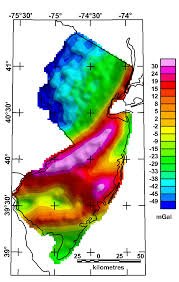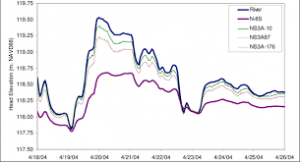## How to Calculate and Solve for Bouguer Anomaly | GravityThe image above represents bourger anomaly.

To compute for bourger anomaly, three essential parameters are needed and these parameters are Free Air Correction (gF), Bouguer Correction (δgB) and Terrain Correction (δgT).

The formula for calculating bourger anomaly:

gB = gF – δgB + δgT

Where:

gB = Bouguer Anomaly
gF = Free Air Correction
δgB = Bouguer Correction
δgT = Terrain Correction

Let’s solve an example;
Find the bourger anomaly when the free air correction is 14, the bourger anomaly is 18 and the terrian correction is 20.

This implies that;

gF = Free Air Correction = 14
δgB = Bouguer Correction = 18
δgT = Terrain Correction = 20

gB = gF – δgB + δgT
gB = 14 – 18 + 20
gB = 14 – 38
gB = -24

Therefore, the bourger anomaly is -24 mGal.

## How to Calculate and Solve for Elevation Correction | GravityThe image above represents elevation correction.

To compute for elevation correction, two essential parameters are needed and these parameters are Free Air Correction (δgFand Bouguer Correction (δgB).

The formula for calculating elevation correction:

δgE = δgF – δgB

Where:

δgE = Elevation Correction
δgF = Free Air Correction
δgB = Bouguer Correction

Let’s solve an example;
Find the elevation correction when the free air correction is 12 and the bourger correction is 17.

This implies that;

δgF = Free Air Correction = 12
δgB = Bouguer Correction = 17

δgE = δgF – δgB
δgE = 12 – 17
δgE = -5

Therefore, the elevation correction is -5 mGal.

Calculating the Free Air Correction when the Elevation Correction and the Bourger Correction is Given.

δgF = δgE + δgB

Where;

δgF = Free Air Correction
δgE = Elevation Correction
δgB = Bouguer Correction

Let’s solve an example;
Find the free air correction when the elevation correction is 34 and the bourger correction is 21.

This implies that;

δgE = Elevation Correction = 34
δgB = Bouguer Correction = 21

δgF = δgE + δgB
δgF = 34 + 21
δgF = 55

Therefore, the free air correction is 55.